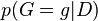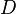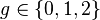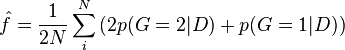ANGSD: Analysis of next generation Sequencing Data

Latest tar.gz version is (0.934/0.935 on github), see Change_log for changes, and download it here.

PostFreq

If the genotype probabilities are known the frequencies can be estimated by summing up the posterior probabilities$p(G=g|D)$ where$D$ is the sequencing data and$g\in\{0,1,2\}$ the allele count of the minor allele. The frequency estimate$\hat{f}=\frac{1}{2N}\sum_i^N \left(2p(G=2|D)+p(G=1|D)\right)$